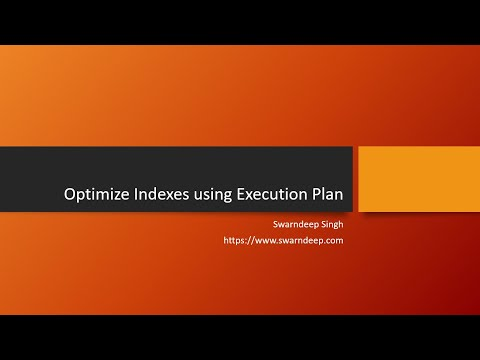## Aug 30, 2010

### Database Backup Growth Report Script

Following Script will return the real-time database backup growth report for Week or Month or Year. Script was developed and tested in SQL 10.0, however, with small changes it can be used in SQL 2000 or SQL 2005.
CREATE proc usp_RPT_Backup_Growth (@dbname varchar(100), @type varchar(100), @servername varchar(100), @year varchar(4), @freq varchar(10))
as
if (@freq='Week')
begin
with bkupreport(database_name, Type, backup_size, server_name, unit_num, year_num) as (
select a.database_name,
case a.type when 'D' then 'Full'
when 'I' then 'Diff'
when 'L' then 'Log' end as Type
,avg(a.compressed_backup_size) Total_Backup_Size
,a.server_name
,DATEPART(wk,a.backup_start_date) 'WeekNumber', DATEPART(year,a.backup_start_date) 'YearNumber'
from msdb.dbo.backupset a with (nOLOCK)
where /*a.backup_start_date between (GETDATE()-32) and GETDATE()
and */a.database_name = @dbname and a.type =@type and a.server_name = @servername
and DATEPART(year,a.backup_start_date) = @year
group by a.database_name, a.type, a.server_name, DATEPART(wk,a.backup_start_date)
, DATEPART(year,a.backup_start_date)
)
select a.database_name, a.Type, a.backup_size, a.server_name, a.unit_num, a.year_num
,Cast((100. * (a.backup_size - b.backup_size)) / b.backup_size As numeric(18,2)) As 'Growth' from bkupreport a
left outer join bkupreport b with (NOLOCK)
on b.unit_num+1 = a.unit_num
order by a.unit_num
end
if (@freq='Month')
begin
with bkupreport(database_name, Type, backup_size, server_name, unit_num, year_num) as (
select a.database_name,
case a.type when 'D' then 'Full'
when 'I' then 'Diff'
when 'L' then 'Log' end as Type
,avg(a.compressed_backup_size) Total_Backup_Size
,a.server_name
,DATEPART(MM,a.backup_start_date) 'MonthNumber', DATEPART(year,a.backup_start_date) 'YearNumber'
from msdb.dbo.backupset a with (nOLOCK)
where /*a.backup_start_date between (GETDATE()-32) and GETDATE()
and */a.database_name = @dbname and a.type =@type and a.server_name = @servername
and DATEPART(year,a.backup_start_date) = @year
group by a.database_name, a.type, a.server_name, DATEPART(MM,a.backup_start_date)
, DATEPART(year,a.backup_start_date)
)
select a.database_name, a.Type, a.backup_size, a.server_name, a.unit_num, a.year_num
,Cast((100. * (a.backup_size - b.backup_size)) / b.backup_size As numeric(18,2)) As 'Growth' from bkupreport a
left outer join bkupreport b with (NOLOCK)
on b.unit_num+1 = a.unit_num
order by a.unit_num
end
if (@freq='Year')
begin
with bkupreport(database_name, Type, backup_size, server_name, unit_num, year_num) as (
select a.database_name,
case a.type when 'D' then 'Full'
when 'I' then 'Diff'
when 'L' then 'Log' end as Type
,avg(a.compressed_backup_size) Total_Backup_Size
,a.server_name
,0 'MonthNumber', DATEPART(year,a.backup_start_date) 'YearNumber'
from msdb.dbo.backupset a with (nOLOCK)
where a.database_name = @dbname and a.type =@type and a.server_name = @servername
group by a.database_name, a.type, a.server_name, DATEPART(year,a.backup_start_date)
, DATEPART(year,a.backup_start_date)
)
select a.database_name, a.Type, a.backup_size, a.server_name, a.unit_num, a.year_num
,Cast((100. * (a.backup_size - b.backup_size)) / b.backup_size As numeric(18,2)) As 'Growth' from bkupreport a
left outer join bkupreport b with (NOLOCK)
on b.year_num+1 = a.year_num
order by a.unit_num
end
GO